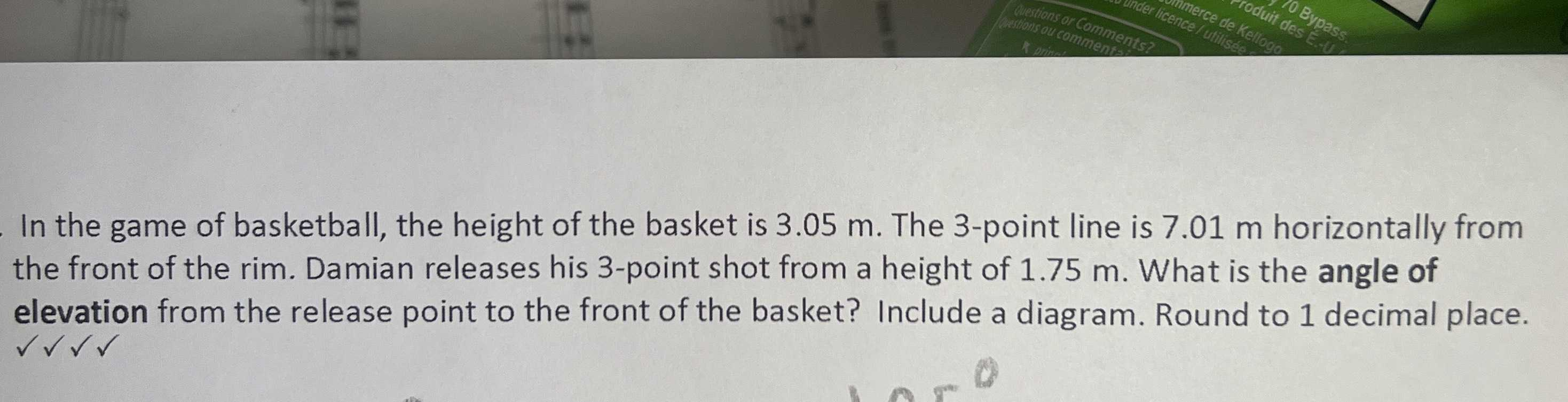### Still have math questions?In the game of basketball, the height of the basket is $$3.05 m$$ . The $$3$$ -point line is $$7.01$$ m horizontally from the front of the rim. Damian releases his $$3$$ -point shot from a height of $$1.75 m$$ . What is the angle of elevation from the release point to the front of the basket? Include a diagram. Round to $$1$$ decimal place.
10.5$$°$$